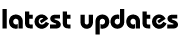# Physics : Motion And Mechanics

KINEMATICS

Kinematics covers a broad range of topics, from bodies falling to earth, to the description of bodies moving in a straight line, to circular motion.

Speed Speed is the ratio of a distance covered by a body in a given amount of time, to that time. It is measured in metres per second.

Velocity velocity is speed measured in a particular direction. Velocity is a vector quantity, which is one in which both the magnitude and direction are stated.

Acceleration and Deceleration

Acceleration is the rate of change of velocity. Acceleration may be defined as the change in velocity over a given time interval. Acceleration is measured in m/s2 (or ms-2).

NEWTON’S LAWS OF MOTION

Newton’s three laws of motion state the fundamental relationships between the acceleration of a body and the forces acting on it.

1. A body will remain stationary or travelling at a constantvelocity unless it is acted upon by an external force. Newton’s first law explains why we lurch forward in a car when it suddenly breaks, and why we are pushed back into our seats when a car suddenly accelerates.

2. The resultant force exerted on a body is directly proportional to the acceleration produced by the force.

The second law of motion can be expressed in an equation: force = mass x acceleration, or F = ma. Forces are measured in newtons. A force of 1 newton will accelerate a mass of 1 kg by 1 m/s2.

3. To every action there is an equal and opposite reaction. When a bullet is fired, thegun recoils backwards. This is caused by a reaction force on the gun from the bullet. From this law can be derived the principle of the conservation of momentum. Momentum, which Newton called the ‘quantity of motion’, is the product of mass and velocity.

NEWTON’S LAW OF GRAVITATION

According to this law every particle in the universe attracts every other particle in the universe. Newton’s Law of Gravitation is thus: F = Gm1m2/r2, where G is the ‘universal gravitational constant’. Further experiments on gravity proved that: G = 6.67206 x 10-11 Nnr-2kg-2.

## No comments

Be the first one to leave a comment.My Great Web page